# 3 linear motion speed and velocity changing velocity

• Slides: 15
Download presentation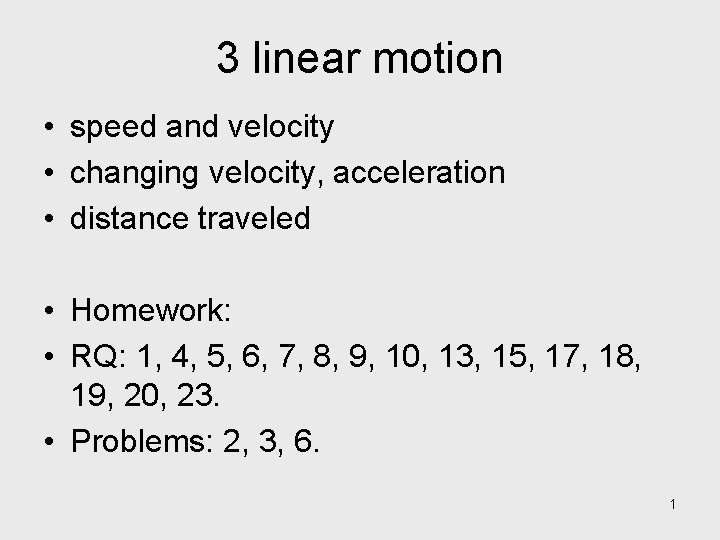3 linear motion • speed and velocity • changing velocity, acceleration • distance traveled • Homework: • RQ: 1, 4, 5, 6, 7, 8, 9, 10, 13, 15, 17, 18, 19, 20, 23. • Problems: 2, 3, 6. 1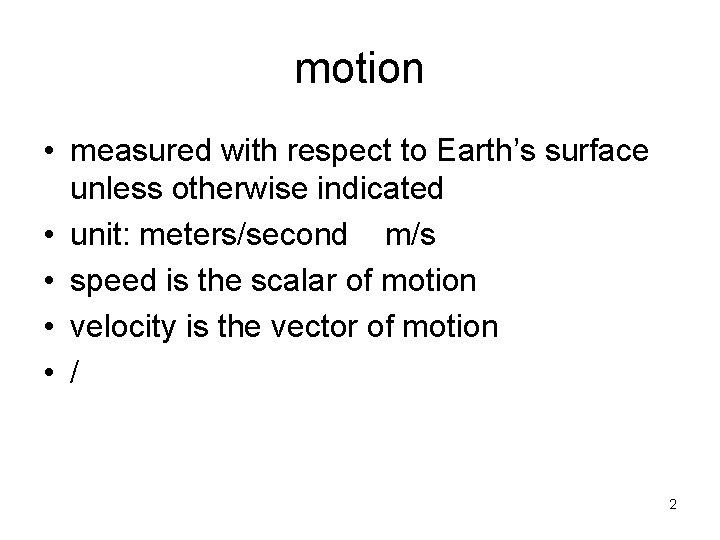motion • measured with respect to Earth’s surface unless otherwise indicated • unit: meters/second m/s • speed is the scalar of motion • velocity is the vector of motion • / 2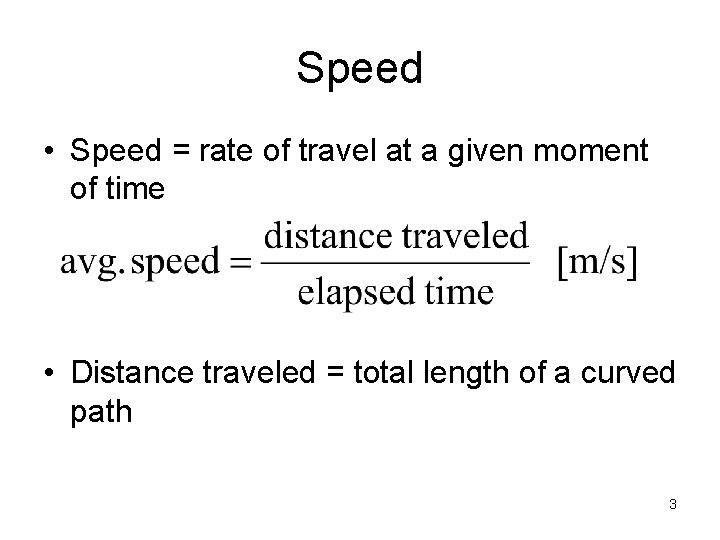Speed • Speed = rate of travel at a given moment of time • Distance traveled = total length of a curved path 3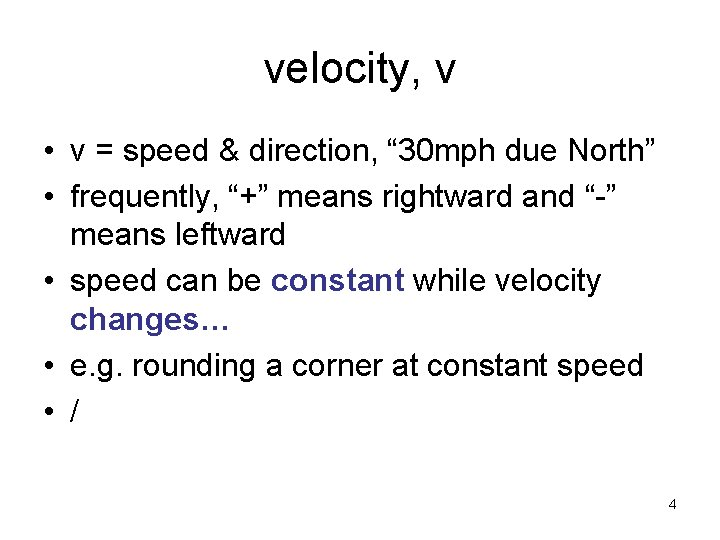velocity, v • v = speed & direction, “ 30 mph due North” • frequently, “+” means rightward and “-” means leftward • speed can be constant while velocity changes… • e. g. rounding a corner at constant speed • / 4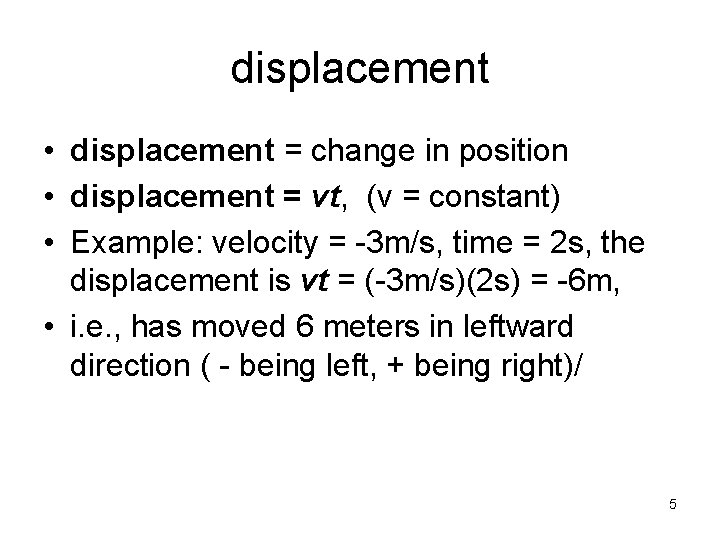displacement • displacement = change in position • displacement = vt, (v = constant) • Example: velocity = -3 m/s, time = 2 s, the displacement is vt = (-3 m/s)(2 s) = -6 m, • i. e. , has moved 6 meters in leftward direction ( - being left, + being right)/ 5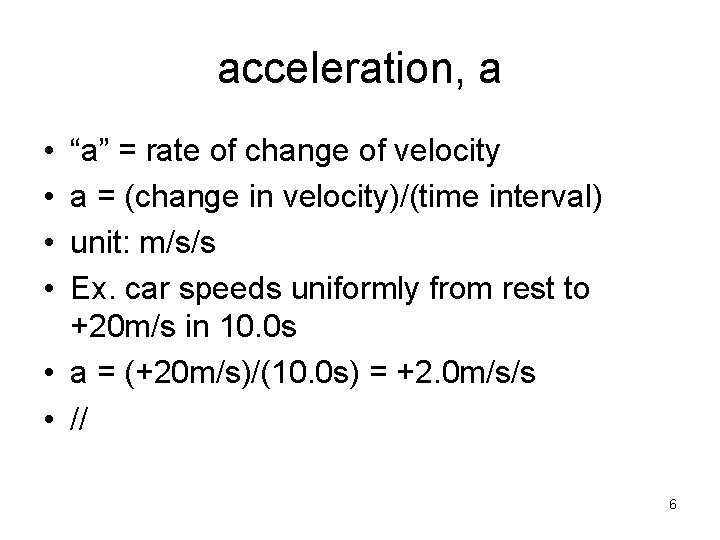acceleration, a • • “a” = rate of change of velocity a = (change in velocity)/(time interval) unit: m/s/s Ex. car speeds uniformly from rest to +20 m/s in 10. 0 s • a = (+20 m/s)/(10. 0 s) = +2. 0 m/s/s • // 6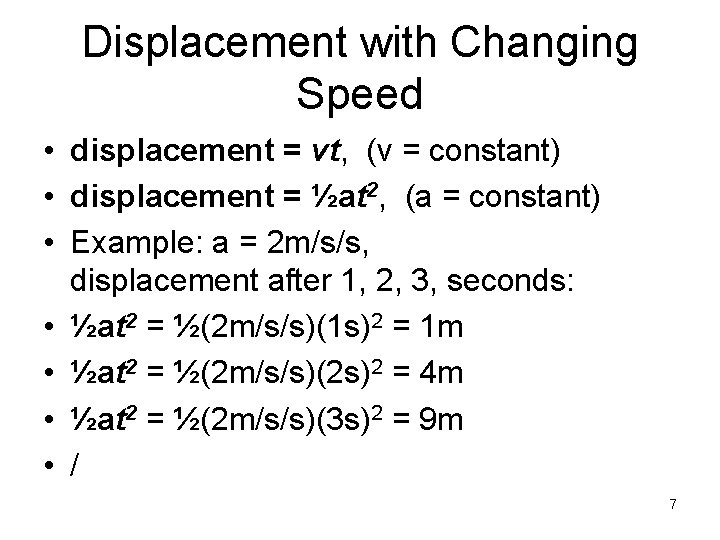Displacement with Changing Speed • displacement = vt, (v = constant) • displacement = ½at 2, (a = constant) • Example: a = 2 m/s/s, displacement after 1, 2, 3, seconds: • ½at 2 = ½(2 m/s/s)(1 s)2 = 1 m • ½at 2 = ½(2 m/s/s)(2 s)2 = 4 m • ½at 2 = ½(2 m/s/s)(3 s)2 = 9 m • / 7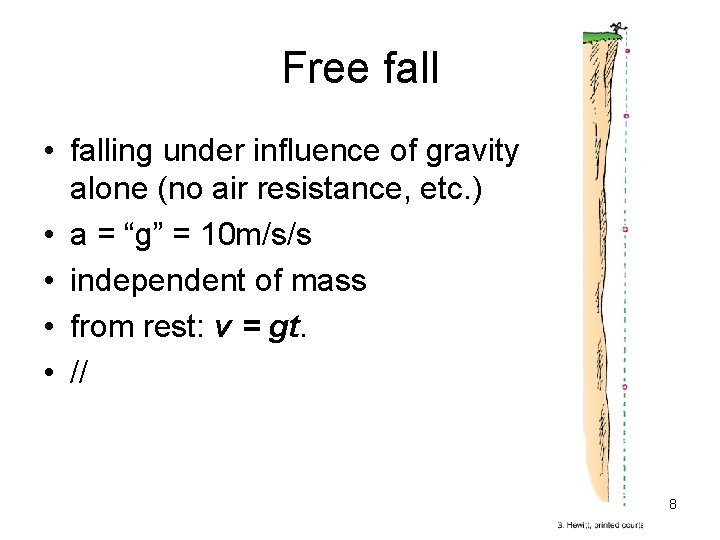Free fall • falling under influence of gravity alone (no air resistance, etc. ) • a = “g” = 10 m/s/s • independent of mass • from rest: v = gt. • // 8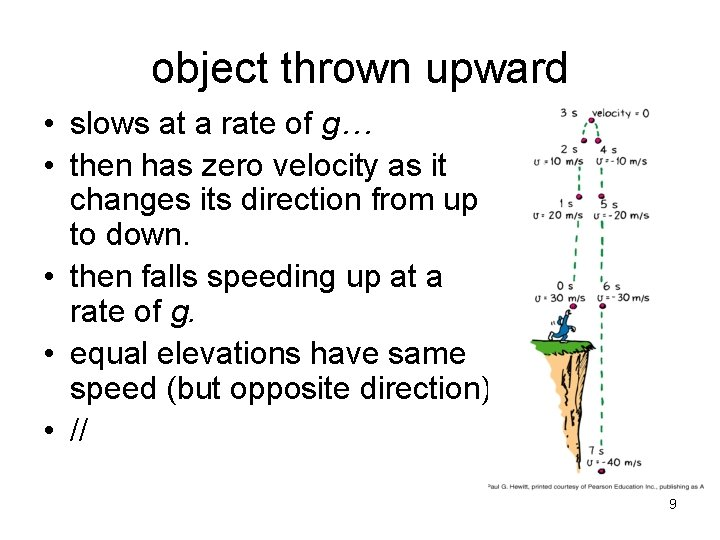object thrown upward • slows at a rate of g… • then has zero velocity as it changes its direction from up to down. • then falls speeding up at a rate of g. • equal elevations have same speed (but opposite direction) • // 9Free-Fall Distance • • initial velocity = 0 final velocity = gt average velocity = ½ (0 + gt) = ½gt. distance d = (average velocity)x(time) d = (½gt)(t) d = ½gt 2. • Example: after 3. 0 seconds: d = ½(10)(3)2 = 5 x 9 = 45 meters • // 10Application: “Hang-time” of jumpers Michael Jordan’s best hang-time was 0. 9 s Round this to 1 s. How high can he jump? Use d = ½ g t 2. For 1 s hang-time, that’s 0. 5 s up and 0. 5 s down. Substituting t = 0. 5 seconds into the distance equation d = ½ (10) (0. 5)2 = 1. 25 m This is about 4 feet! 113 summary • • • speed = rate of travel velocity = speed and direction acceleration = rate of change of velocity General: v = at d = ½at 2. for free-fall: a = g = 10 m/s/s 123 agenda • lecture • practicing physics: p 8, p 10 • lab: measurement of constant velocity and constant acceleration 13Question (to think about…) 14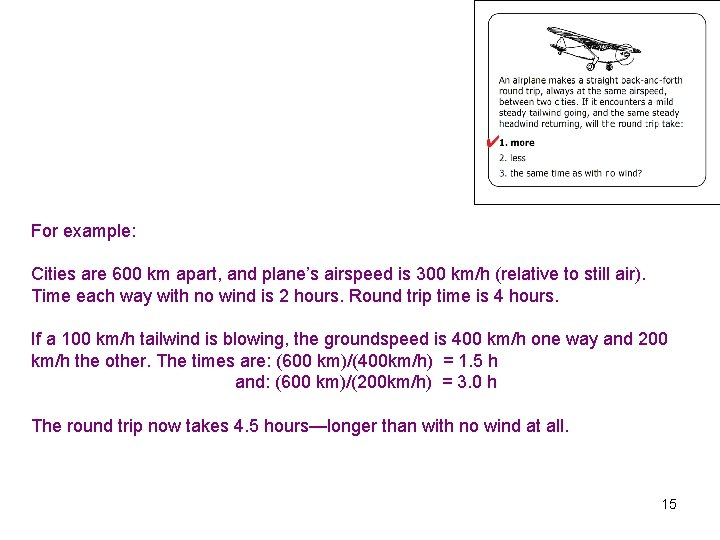For example: Cities are 600 km apart, and plane’s airspeed is 300 km/h (relative to still air). Time each way with no wind is 2 hours. Round trip time is 4 hours. If a 100 km/h tailwind is blowing, the groundspeed is 400 km/h one way and 200 km/h the other. The times are: (600 km)/(400 km/h) = 1. 5 h and: (600 km)/(200 km/h) = 3. 0 h The round trip now takes 4. 5 hours—longer than with no wind at all. 15